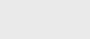4个月前 (05-14) |   抢沙发  231Java中的流实现在默认情况下是顺序的，除非在并行中显式地提到它。当一个流以并行方式执行时，Java运行时将该流划分为多个子流。聚合操作通过并行迭代这些子流来处理这些子流，然后合并这些结果。因此，并行流可用于顺序流具有性能影响的区域

``````private long countPrimes(int max) {
return range(1, max).parallel().filter(this::isPrime).count();
}

private boolean isPrime(long n) {
return n > 1 && rangeClosed(2, (long) sqrt(n)).noneMatch(divisor -> n % divisor == 0);
}``````

``````private List<StockInfo> getStockInfo(Stream<String> symbols) {
return symbols.parallel()
.map(this::getStockInfo) //slow network operation
.collect(toList());
}``````

``````private void run() throws InterruptedException {
// Simulating multiple threads in the system
// if one of them is executing a long-running task.
// for it to finish
es.execute(() -> countPrimes(MAX, 1000));
es.execute(() -> countPrimes(MAX, 0));
es.execute(() -> countPrimes(MAX, 0));
es.execute(() -> countPrimes(MAX, 0));
es.execute(() -> countPrimes(MAX, 0));
es.execute(() -> countPrimes(MAX, 0));
es.shutdown();
es.awaitTermination(60, TimeUnit.SECONDS);
}

private void countPrimes(int max, int delay) {
System.out.println(range(1, max).parallel().filter(this::isPrime).peek(i -> sleep(delay)).count());
}``````### 注册